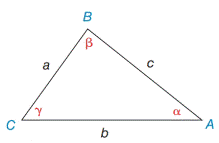Chapter 11.4, Problem 8EElementary Geometry For College St...

7th Edition
Alexander + 2 others
ISBN: 9781337614085

Solutions

Chapter
SectionElementary Geometry For College St...

7th Edition
Alexander + 2 others
ISBN: 9781337614085
Textbook Problem

In Exercises 7 and 8, state the form of the Law of Sines or the Law of Cosines that you would use to solve the problem. See the figure for Exercises 1 to 8.a) Find b if you know the values of a, c, and β .b) Find b if you know the values of a, α , and β .Exercises 1-8

To determine

a.

To Find:

The side b with the side a, c, and the angle β.

Explanation

Formula:

The Law of Cosines

a2=b2+c22bccosα

b2=a2+c22accosβ

c2=a2+b22abcosγ

Where α, β, and γ is the acute angle of the triangle, and a, b, and c is the sides of the triangle.

Calculation:

Given,

The dimensions area a, c and the angle β of the triangle ABC

To determine

b.

To Find:

The side b with the angles αβ with side a

Still sussing out bartleby?

Check out a sample textbook solution.

See a sample solution

The Solution to Your Study Problems

Bartleby provides explanations to thousands of textbook problems written by our experts, many with advanced degrees!

Get Started